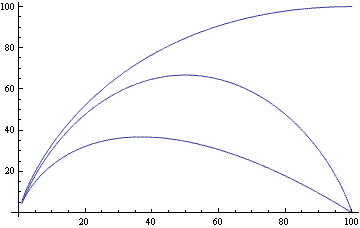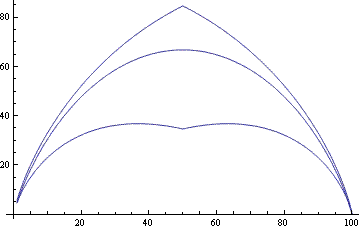# Upper and lower bounds on binomial coefficients

Binomial coefficients are easy to motivate and define. In its simplest form, the binomial coefficient (n, k) is the number of ways to choose k things from a set of n things and equals n!/(k! (nk)!). On the other hand, binomial coefficients can be hard to work with when proving theorems.

In applications, such as establishing bounds on an algorithm’s run time, it’s often enough to have upper bound or lower bounds on binomial coefficients that are easier to work with. Here’s a pair of such bounds.for positive integers n and k with 1 ≤ kn.

The bounds above are easy to remember due to the typographical similarity between the three terms. Start with the binomial coefficient symbol. To get a lower bound, add a horizontal bar to turn the coefficient into a fraction and add an exponent of k. To get an upper bound, insert a factor of “e” inside the lower bound.

I found the bounds above in Introduction to Algorithms. The statement and proof were limited to integer arguments, but binomial coefficients can be defined more generally and the bounds appear to be valid for real values of n and k, not just integer values.

How good are the bounds? Here’s a plot of the upper and lower bounds as well as the true value. Because binomial coefficients can get very large, I plotted the logarithms of the bounds and true values. In this plot n = 100 and k varies between 1 and 100 (including non-integer values).The lower bound is exact at the left end and the right end and is worse in the middle.

The upper bound is quite good for small k but terrible for large k. However, we could easily improve the upper bound by using the fact that binomial(n, k) = binomial(n, nk). For k larger than n/2, we could apply the upper bound to nk rather than k. We could do the same for the lower bound, though the benefit in doing so is not as great.

Here’s the plot that uses the best upper and lower bounds switching from binomial(n, k) to binomial(nk) for k > n/2.I experimented briefly with other values of n and got very similar plots.

Related post: How to compute binomial coefficients

## 5 thoughts on “Upper and lower bounds on binomial coefficients”

1. The lower bound is, of course, seems to be more precise, because it is off by approx sqrt(n). What I find more useful, however, is an estimate for upper/lower tails. In that, Hoeffding’s inequality seems to be very useful. Cormen et al apparently are deriving even more tighter bounds.

2. PS: tails of the binomial distribution.

3. It sort of looks like Stirling’s equivalent of n! Is it related in any way ?

4. Cool! Thanks! just what I needed (mainly the upper bound)

5. random_guy

The upper bound is actually useless around k = n/2, so we can make it even better, upper bounding by 2^(n). For n = 100, this would correspond to ln(2^100) ~ 69.31.